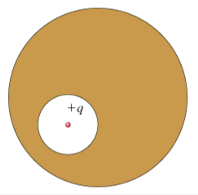# Problem: A spherical cavity is hollowed out of the interior of a neutral conducting sphere. At the center of the cavity is a point charge, of positive charge q.Now a second charge, q2, is brought near the outside of the conductor. Which of the following quantities would change?(a) The electric cavity within the cavity, Ecav.would changewould not change(b) The electric field outside the conductor, Eext.would changewould not change

###### FREE Expert Solution

Gauss law:

$\overline{){{\mathbf{\varphi }}}_{{\mathbf{E}}}{\mathbf{=}}{\mathbf{\oint }}{\mathbf{E}}{\mathbf{·}}{\mathbf{d}}{\mathbf{A}}{\mathbf{=}}\frac{{\mathbf{q}}_{\mathbf{enc}}}{{\mathbf{\epsilon }}_{\mathbf{0}}}}$

(a)

The charge enclosed the cavity has not changed. Hence, the flux will not change.###### Problem Details

A spherical cavity is hollowed out of the interior of a neutral conducting sphere. At the center of the cavity is a point charge, of positive charge q.Now a second charge, q2, is brought near the outside of the conductor. Which of the following quantities would change?

(a) The electric cavity within the cavity, Ecav.

• would change
• would not change

(b) The electric field outside the conductor, Eext.

• would change
• would not change